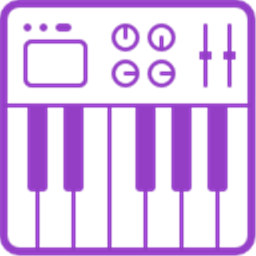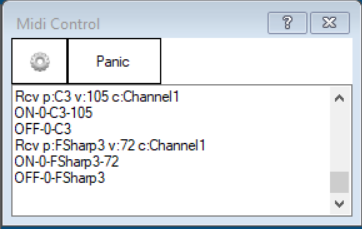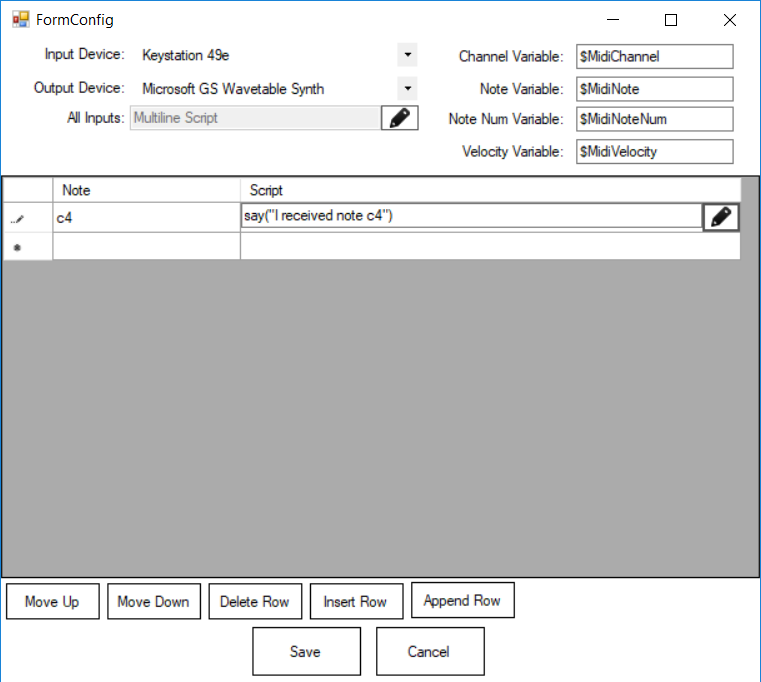• Overview
• Artificial Intelligence
• Audio
• Camera
• Digital
• Games
• General
• Gps
• Graphs
• Hbridge
• I2c
• Infrared Distance
• Machine Learning
• Misc
• Mobile
• Pwm
• Rafiki
• Scripting
• Servo
• Third Party Robots
• Touch Tablet
• Ultrasonic Distance
• Virtual Reality## Midi Control

4. The installer will add this control to EZ-Builder.
6. Choose the Audio category tab.
7. Press the Midi Control icon to add the control to your project.

Send or receive MIDI messages from musical instruments or controllers. EZ-Script can be added to each received note in the configuration window. Use the ControlCommand() to send MIDI messages to instruments.

Any computer with a soundcard will also have a Midi device, usually called Microsoft GS Wave Table Synth, which will output music instrument from the soundcard output. Otherwise, if you have an external midi device, such as a drum machine or keyboard, that can be selected as well.

The status window displays the activity of notes being received from the MIDI device and notes being transmitted.The configuration window of this plugin has many settings. You can configure a script to execute on specific notes. Or, you can have a global script which executes on all other notes. The variables are populated with the most recent incoming note event.Example #1
This example demonstrates how to output random notes within a random delay. Add this to an EZ-Script control and press START, once the MIDI plugin has been configured for a correct output midi device.

#### `Code:`

``:repeat\$rand = getRandom(50, 70)ControlCommand("Midi Control", "SendNote", 1, \$rand, 127, 2000)sleepRandom(500, 2000)goto(repeat)``

Example #2
If you add this code to the All Note Events code in the configuration screen, the computer will speak every note that is pressed on the MIDI input keyboard. For example, if you press note C4 on the musical MIDI keyboard, the computer will speak "I received note C4". The computer will also output the note to the MIDI output device.
`Code:say("I received note " + \$MidiNote)ControlCommand("Midi Control", SendNote, \$MidiChannel, \$MidiNote, \$MidiVelocity, 1000)`

Notes Pitch List
You can specify either the string (i.e. C4) or numerical value (i.e. 60) in the ControlCommand().

//
// Summary:
// C in octave -1.
CNeg1 = 0,
//
// Summary:
// C# in octave -1.
CSharpNeg1 = 1,
//
// Summary:
// D in octave -1.
DNeg1 = 2,
//
// Summary:
// D# in octave -1.
DSharpNeg1 = 3,
//
// Summary:
// E in octave -1.
ENeg1 = 4,
//
// Summary:
// F in octave -1.
FNeg1 = 5,
//
// Summary:
// F# in octave -1.
FSharpNeg1 = 6,
//
// Summary:
// G in octave -1.
GNeg1 = 7,
//
// Summary:
// G# in octave -1.
GSharpNeg1 = 8,
//
// Summary:
// A in octave -1.
ANeg1 = 9,
//
// Summary:
// A# in octave -1.
ASharpNeg1 = 10,
//
// Summary:
// B in octave -1.
BNeg1 = 11,
//
// Summary:
// C in octave 0.
C0 = 12,
//
// Summary:
// C# in octave 0.
CSharp0 = 13,
//
// Summary:
// D in octave 0.
D0 = 14,
//
// Summary:
// D# in octave 0.
DSharp0 = 15,
//
// Summary:
// E in octave 0.
E0 = 16,
//
// Summary:
// F in octave 0.
F0 = 17,
//
// Summary:
// F# in octave 0.
FSharp0 = 18,
//
// Summary:
// G in octave 0.
G0 = 19,
//
// Summary:
// G# in octave 0.
GSharp0 = 20,
//
// Summary:
// A in octave 0.
A0 = 21,
//
// Summary:
// A# in octave 0, usually the lowest key on an 88-key keyboard.
ASharp0 = 22,
//
// Summary:
// B in octave 0.
B0 = 23,
//
// Summary:
// C in octave 1.
C1 = 24,
//
// Summary:
// C# in octave 1.
CSharp1 = 25,
//
// Summary:
// D in octave 1.
D1 = 26,
//
// Summary:
// D# in octave 1.
DSharp1 = 27,
//
// Summary:
// E in octave 1.
E1 = 28,
//
// Summary:
// F in octave 1.
F1 = 29,
//
// Summary:
// F# in octave 1.
FSharp1 = 30,
//
// Summary:
// G in octave 1.
G1 = 31,
//
// Summary:
// G# in octave 1.
GSharp1 = 32,
//
// Summary:
// A in octave 1.
A1 = 33,
//
// Summary:
// A# in octave 1.
ASharp1 = 34,
//
// Summary:
// B in octave 1.
B1 = 35,
//
// Summary:
// C in octave 2.
C2 = 36,
//
// Summary:
// C# in octave 2.
CSharp2 = 37,
//
// Summary:
// D in octave 2.
D2 = 38,
//
// Summary:
// D# in octave 2.
DSharp2 = 39,
//
// Summary:
// E in octave 2.
E2 = 40,
//
// Summary:
// F in octave 2.
F2 = 41,
//
// Summary:
// F# in octave 2.
FSharp2 = 42,
//
// Summary:
// G in octave 2.
G2 = 43,
//
// Summary:
// G# in octave 2.
GSharp2 = 44,
//
// Summary:
// A in octave 2.
A2 = 45,
//
// Summary:
// A# in octave 2.
ASharp2 = 46,
//
// Summary:
// B in octave 2.
B2 = 47,
//
// Summary:
// C in octave 3.
C3 = 48,
//
// Summary:
// C# in octave 3.
CSharp3 = 49,
//
// Summary:
// D in octave 3.
D3 = 50,
//
// Summary:
// D# in octave 3.
DSharp3 = 51,
//
// Summary:
// E in octave 3.
E3 = 52,
//
// Summary:
// F in octave 3.
F3 = 53,
//
// Summary:
// F# in octave 3.
FSharp3 = 54,
//
// Summary:
// G in octave 3.
G3 = 55,
//
// Summary:
// G# in octave 3.
GSharp3 = 56,
//
// Summary:
// A in octave 3.
A3 = 57,
//
// Summary:
// A# in octave 3.
ASharp3 = 58,
//
// Summary:
// B in octave 3.
B3 = 59,
//
// Summary:
// C in octave 4, also known as Middle C.
C4 = 60,
//
// Summary:
// C# in octave 4.
CSharp4 = 61,
//
// Summary:
// D in octave 4.
D4 = 62,
//
// Summary:
// D# in octave 4.
DSharp4 = 63,
//
// Summary:
// E in octave 4.
E4 = 64,
//
// Summary:
// F in octave 4.
F4 = 65,
//
// Summary:
// F# in octave 4.
FSharp4 = 66,
//
// Summary:
// G in octave 4.
G4 = 67,
//
// Summary:
// G# in octave 4.
GSharp4 = 68,
//
// Summary:
// A in octave 4.
A4 = 69,
//
// Summary:
// A# in octave 4.
ASharp4 = 70,
//
// Summary:
// B in octave 4.
B4 = 71,
//
// Summary:
// C in octave 5.
C5 = 72,
//
// Summary:
// C# in octave 5.
CSharp5 = 73,
//
// Summary:
// D in octave 5.
D5 = 74,
//
// Summary:
// D# in octave 5.
DSharp5 = 75,
//
// Summary:
// E in octave 5.
E5 = 76,
//
// Summary:
// F in octave 5.
F5 = 77,
//
// Summary:
// F# in octave 5.
FSharp5 = 78,
//
// Summary:
// G in octave 5.
G5 = 79,
//
// Summary:
// G# in octave 5.
GSharp5 = 80,
//
// Summary:
// A in octave 5.
A5 = 81,
//
// Summary:
// A# in octave 5.
ASharp5 = 82,
//
// Summary:
// B in octave 5.
B5 = 83,
//
// Summary:
// C in octave 6.
C6 = 84,
//
// Summary:
// C# in octave 6.
CSharp6 = 85,
//
// Summary:
// D in octave 6.
D6 = 86,
//
// Summary:
// D# in octave 6.
DSharp6 = 87,
//
// Summary:
// E in octave 6.
E6 = 88,
//
// Summary:
// F in octave 6.
F6 = 89,
//
// Summary:
// F# in octave 6.
FSharp6 = 90,
//
// Summary:
// G in octave 6.
G6 = 91,
//
// Summary:
// G# in octave 6.
GSharp6 = 92,
//
// Summary:
// A in octave 6.
A6 = 93,
//
// Summary:
// A# in octave 6.
ASharp6 = 94,
//
// Summary:
// B in octave 6.
B6 = 95,
//
// Summary:
// C in octave 7.
C7 = 96,
//
// Summary:
// C# in octave 7.
CSharp7 = 97,
//
// Summary:
// D in octave 7.
D7 = 98,
//
// Summary:
// D# in octave 7.
DSharp7 = 99,
//
// Summary:
// E in octave 7.
E7 = 100,
//
// Summary:
// F in octave 7.
F7 = 101,
//
// Summary:
// F# in octave 7.
FSharp7 = 102,
//
// Summary:
// G in octave 7.
G7 = 103,
//
// Summary:
// G# in octave 7.
GSharp7 = 104,
//
// Summary:
// A in octave 7.
A7 = 105,
//
// Summary:
// A# in octave 7.
ASharp7 = 106,
//
// Summary:
// B in octave 7.
B7 = 107,
//
// Summary:
// C in octave 8, usually the highest key on an 88-key keyboard.
C8 = 108,
//
// Summary:
// C# in octave 8.
CSharp8 = 109,
//
// Summary:
// D in octave 8.
D8 = 110,
//
// Summary:
// D# in octave 8.
DSharp8 = 111,
//
// Summary:
// E in octave 8.
E8 = 112,
//
// Summary:
// F in octave 8.
F8 = 113,
//
// Summary:
// F# in octave 8.
FSharp8 = 114,
//
// Summary:
// G in octave 8.
G8 = 115,
//
// Summary:
// G# in octave 8.
GSharp8 = 116,
//
// Summary:
// A in octave 8.
A8 = 117,
//
// Summary:
// A# in octave 8.
ASharp8 = 118,
//
// Summary:
// B in octave 8.
B8 = 119,
//
// Summary:
// C in octave 9.
C9 = 120,
//
// Summary:
// C# in octave 9.
CSharp9 = 121,
//
// Summary:
// D in octave 9.
D9 = 122,
//
// Summary:
// D# in octave 9.
DSharp9 = 123,
//
// Summary:
// E in octave 9.
E9 = 124,
//
// Summary:
// F in octave 9.
F9 = 125,
//
// Summary:
// F# in octave 9.
FSharp9 = 126,
//
// Summary:
// G in octave 9.
G9 = 127